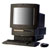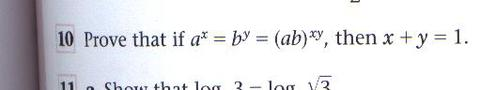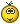# Any help on this problem? (Maths)

2 posts / 0 newOffline
Last seen: 11 years 9 months ago
Joined: Dec 20 2003 - 10:38
Posts: 634
Any help on this problem? (Maths)

All I manage to do is show that X=X! Hardly ground breaking...Any solutions??Thanks

Offline
Last seen: 9 years 8 months ago
Joined: Dec 20 2003 - 10:38
Posts: 70
I'll take a stab at it...

Why don't you show us your work? Maybe someone can see where your error is.

Did you start with three separate simultaneous equations? Did you CORRECTLY isolate for x by adding or subtracting two of the three equations and applying the log function? Did you CORRECTLY isolate for y by adding or subtracting two of the three equations and applying the log function? ARE YOU APPLYING THE LOG FUNCTION CORRECTLY? Did you add the new equations together to create an x + y expression within an equation? Were you able to isolate the x + y expression? Were you able to manipulate the remaining algabraic expressions so that x + y = 1 ? Q.E.D. if you did.

tony b.